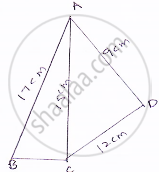# Find the Perimeter and Area of the Quadrilateral Abcd in Which Ab = 17 Cm, Ad =9 Cm, Cd = L2cm, ∠Acb = 90° and Ac=L5cm. - Mathematics

Find the perimeter and area of the quadrilateral ABCD in which AB = 17 cm, AD =9 cm, CD = l2cm, ∠ACB = 90° and AC=l5cm.

#### Solution

The sides of a quadrilateral ABCD in which AB = 17 cm, AD = 9 cm, CD = 12 cm, ∠ACB = 90° and AC = 15 cm
Here, By using Pythagoras theorem

BC=sqrt(17^2-15^2) = sqrt(289-225)=sqrt64=8cm

Now, area of ΔABC=1/2xx8xx15=60cm^2

For area of Δle ACD,
Let a = 15 cm, b = 12 cm and c = 9 cm

Therefore, S =(15+12+9)/2=36/2=18cmArea of ACD =sqrt(s(s-a)(s-b)(s-c))

=sqrt(18(18-15)(18-12)(18-9))

=sqrt(18xx18xx3xx3)

=sqrt((18xx3)^2)

=54cm^2

∴ Thus, the area of quadrilateral ABCD = 60 + 54 = 114 cm^2

Concept: Application of Heron’s Formula in Finding Areas of Quadrilaterals
Is there an error in this question or solution?

#### APPEARS IN

RD Sharma Mathematics for Class 9
Chapter 17 Heron’s Formula
Exercise 17.2 | Q 13 | Page 20

Share